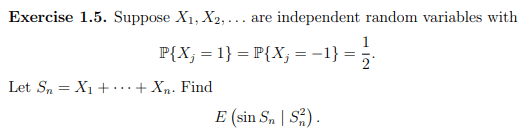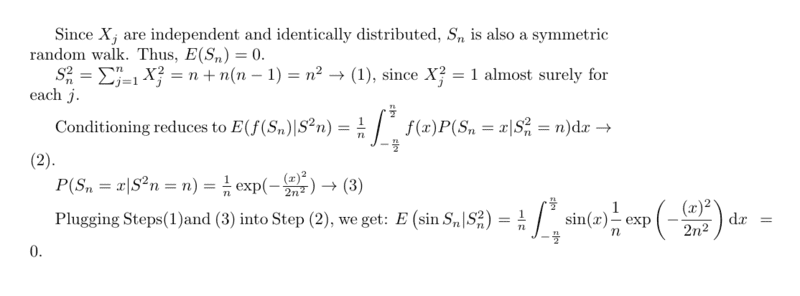# Finding expectation of the function of a sum of i.i.d. random variables

• I
WMDhamnekar
MHB
TL;DR Summary
Finding expectation of the function of a sum of i.i.d. random variables given the 2nd moment of sum of i. i. d. random variables.##S_n has a discrete distribution. How did you get continuous?

•WMDhamnekar
WMDhamnekar
MHB
Oh, I am sorry. You are correct. So, if ## S_n## has a discrete distribution, my answer would be
* ##S_n## is a random walk with symmetric steps, so ##E(S_n) = 0##
* ##S_n^2## is the sum of n independent random variables taking values 1 and -1 with equal probability, so ##E(S_n^2) = n##
* By conditional expectation, ##E(\sin{S_n} | S_n^2) = E(\sin{S_n} | n) = \frac{E(\sin{S_n})}{E(n)} = \frac{0}{n} = 0##.

Last edited:
Staff Emeritus
Gold Member
I don't think you're handling the conditional part right. Your final answer is supposed to be a function of ##S_n^2## - given a specific value that it ends up being, what is the expected value of the sine?

WMDhamnekar
MHB
I don't think you're handling the conditional part right. Your final answer is supposed to be a function of ##S_n^2## - given a specific value that it ends up being, what is the expected value of the sine?
I edited my answer to this question. Does it look now correct?

Staff Emeritus
Gold Member
No, I still think you have written meaningless notation.

Try to compute ##E(\sin(S_n) | S_n)## what does this even mean?

WMDhamnekar
MHB
No, I still think you have written meaningless notation.

Try to compute ##E(\sin(S_n) | S_n)## what does this even mean?
##S_n= \pm\sqrt{n}## with equal probability ##\frac12 \therefore E(\sin{(S_n)}|S^2_n)=\sin{(S_n=0)}\times \frac12 - \sin{(S_n=0)}\times \frac12 =0##

Staff Emeritus